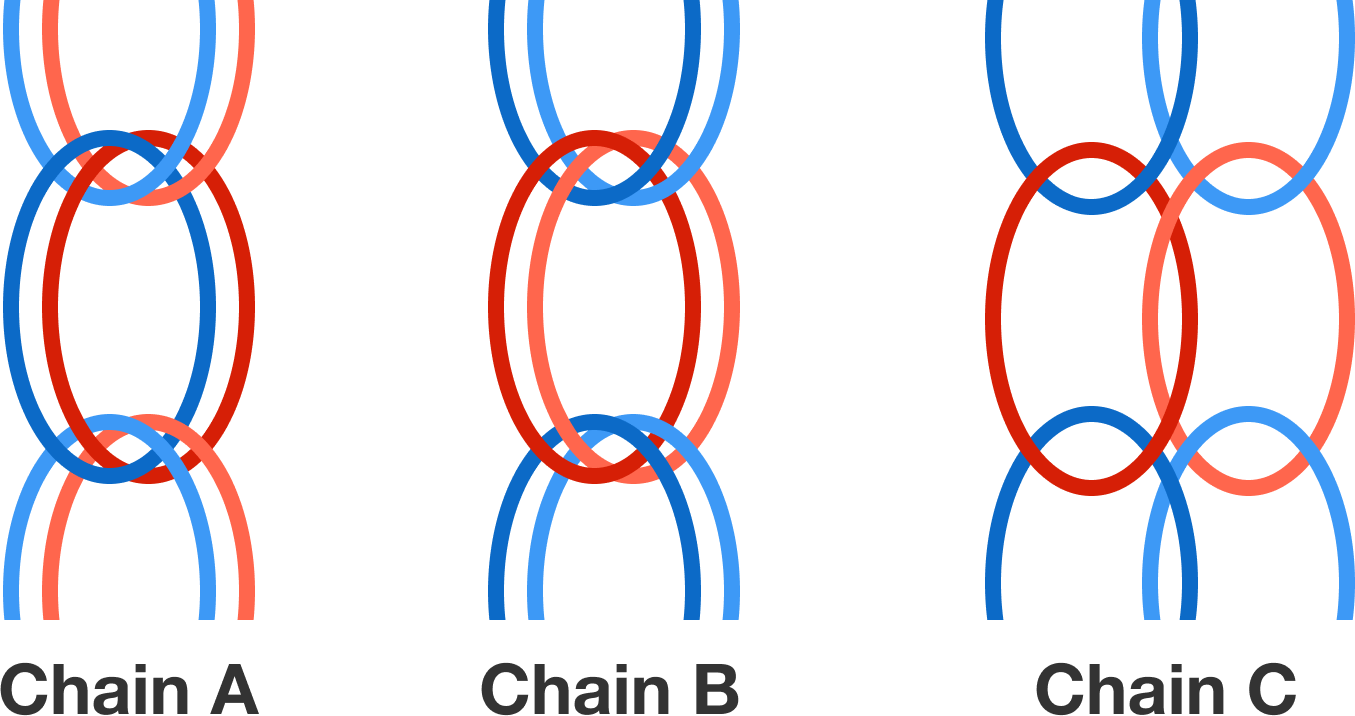# Rubber Physics (Part 1)

Amy makes bracelets out of colorful rubber bands. Playing around with the rubber, she notices that the red rubber bands are much stiffer than the blue ones. To get uniformly elastic bracelets, she tries to combine red and blue bands in equal parts. As a first step, she makes three different types of chains from the rubber bands outlined in the figure. However, while trying out the chains, Amy is surprised to find that one chain is much stiffer than the other two. Which rubber chain is it?Hint: For small displacements $x$, a rubber band obeys Hook's law $f = - k x$ with reversing force $f$ and the force constant $k$. Figure out which force constant $k$ results when two different rubber bands with force constants $k_1$ and $k_2$ are linked in parallel or in series. (The chaining of rubber bands resembles the parallel and series connection in an electrical circuit.)

×